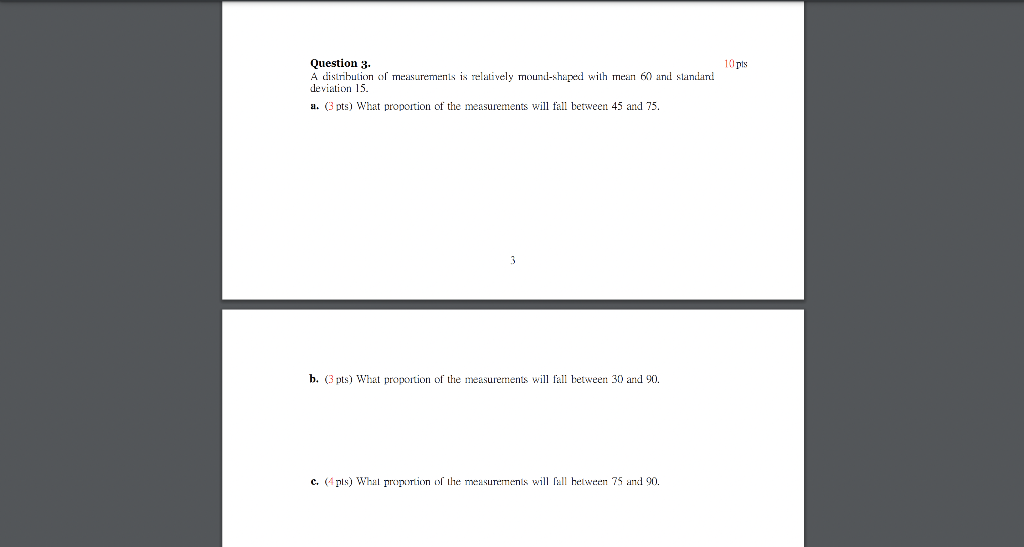# Question Solved1 Answer Question 3. 10 pts A distribution of measurements is relatively mound-shaped with mean 60 and standard deviation 15. a. (3 pts) What proportion of the measurements will fall between 45 and 75. b. (3 pts) What proportion of the measurements will fall between 30 and 90. c. (4 pts) What proportion of the measurements will fall between 75 and 90. Question 3. 10 pts A distribution of measurements is relatively mound-shaped with mean 60 and standard deviation 15. a. (3 pts) What proportion of the measurements will fall between 45 and 75. b. (3 pts) What proportion of the measurements will fall between 30 and 90. c. (4 pts) What proportion of the measurements will fall between 75 and 90.TTSTJH The Asker · Probability and StatisticsTranscribed Image Text: Question 3. 10 pts A distribution of measurements is relatively mound-shaped with mean 60 and standard deviation 15. a. (3 pts) What proportion of the measurements will fall between 45 and 75. b. (3 pts) What proportion of the measurements will fall between 30 and 90. c. (4 pts) What proportion of the measurements will fall between 75 and 90.
More
Transcribed Image Text: Question 3. 10 pts A distribution of measurements is relatively mound-shaped with mean 60 and standard deviation 15. a. (3 pts) What proportion of the measurements will fall between 45 and 75. b. (3 pts) What proportion of the measurements will fall between 30 and 90. c. (4 pts) What proportion of the measurements will fall between 75 and 90.